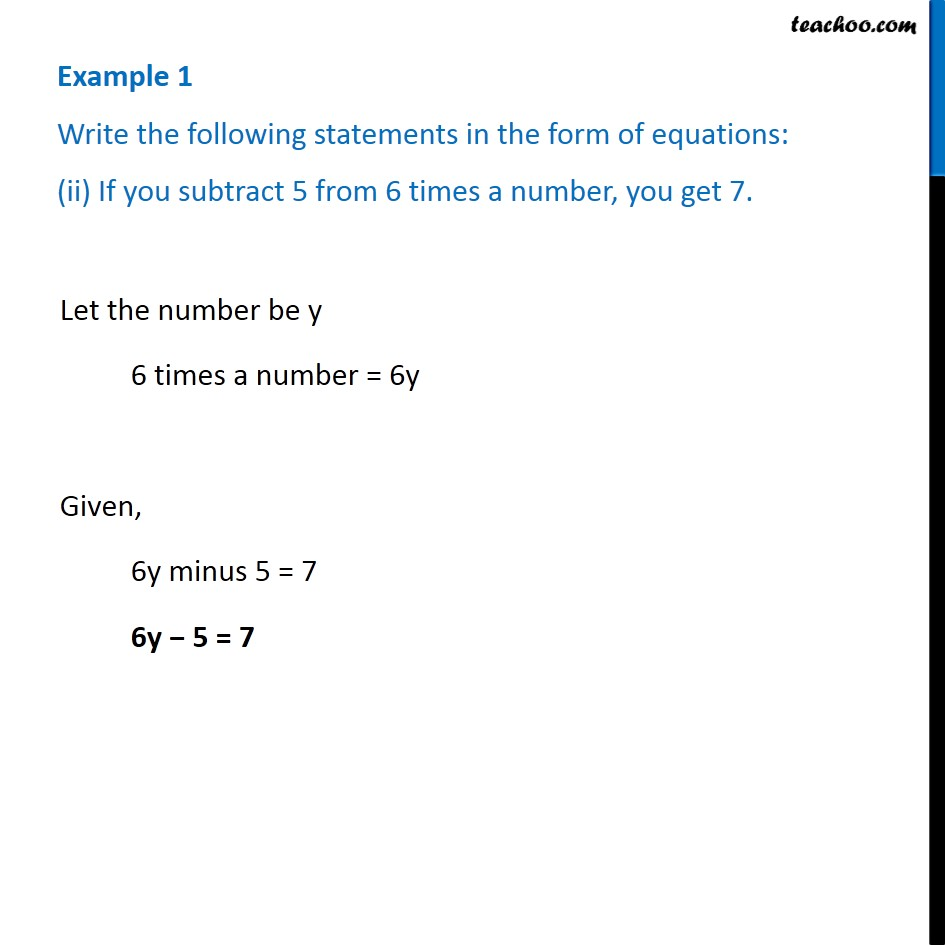Examples

Chapter 4 Class 7 Simple Equations
Serial order wiseLearn in your speed, with individual attention - Teachoo Maths 1-on-1 Class

### Transcript

Example 1 Write the following statements in the form of equations: (ii) If you subtract 5 from 6 times a number, you get 7.Let the number be y 6 times a number = 6y Given, 6y minus 5 = 7 6y − 5 = 7